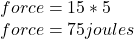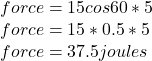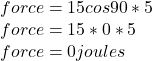Question

Calculate the transfer of energy by a force of 15N when it movies the object by a distance of 5 m
a. In the direction of the force
b. In the direction at 60 degrees to the direction of the force
c. At right angles to the force

1.a. 75 joules

b. 37.5 joules

c. 0 joules

Explanation:

work is defined as the product of force times the distance moved in the direction of the force

Given data

force F= 15N

distance S= 5m

1. In the direction of the force2. In the direction at 60 degrees to the direction of the force3.  At right angles to the force